# ANOVA

Analysis of variance tests differences among means by analyzing variance.

TestDVNumber of GroupsType of Group
One Sample t-TestCompares meansCompares a sample to the populationPopulation
Two Sample independent t-TestCompares meansCompares two samplesIndependent (unrelated)
Two sample dependent t-TestCompares meansCompares two samplesDependent (related)
Between groups ANOVACompares meansCompares two or more levels of an IVIndependent (unrelated)
Repeated Measure ANOVACompares meansCompares two or more levels of an IVDependent (related)
Mann-Whitney URanked data or Not normally distributedCompares two groupsIndependent (unrelated)
Wilcoxon Match-Pair Signed-Rank testRanked data or Not normally distributedCompares two groupsDepended (related)
Kruskal-Wallis One-Way ANOVARanked data or Not normally distributedCompares two or more groupsIndependent (unrelated)
Friedman’s testRanked data or Not normally distributedCompares two or more groupsDependent (related)

IV: independent variable

DV: dependent variable

## One Way ANOVA#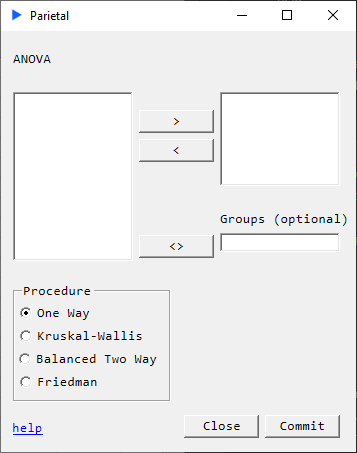#### Description#

Parametric test to compare means of multiple (three or more) samples.

$H_{0}$ : the central values of all data distributions are equal
$H_{a}$ : at least one of the central values is different

#### Assumptions:#

• Dependent variables (more accurately its residuals) are normally distributed
• Variances are homogenous
• Independent samples

#### Returns#

SourceSSDFMSFP
$Treatment\hspace{1mm}$$SST\hspace{3mm}$$k-1\hspace{3mm}$$SST/(k-1)\hspace{3mm}$$MST/MSE\hspace{3mm}$$p Error\hspace{1mm}$$SSE\hspace{3mm}$$N-k\hspace{3mm}$$SSE/(N-k)\hspace{3mm}$
$Total\hspace{1mm}$$SS\hspace{3mm}$$N-1\hspace{3mm}$

### Example#

The following dataset is taken from Introduction to Statistics by Lane et al.

##### Data Format 1:#

Suppose our dataset is of the form:

Group 1Group 2Group 3
328
445
565

We can run a one-way anova using: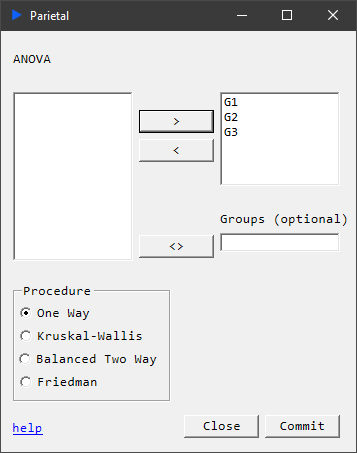This produces the following table: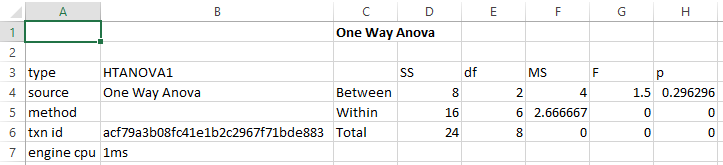##### Data Format 2:#

Another way of formatting the table for analysis is as follows. The advantage of this is that the number of elements per group do not have to be the same.

GroupY
13
14
15
22
24
26
38
35
35This produces the following table: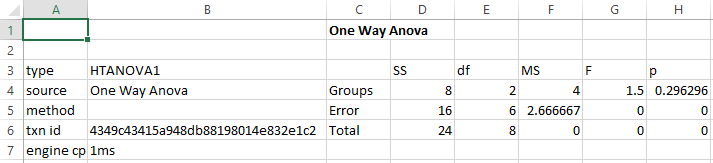Example Reference: One-Factor ANOVA. (2021, January 10). Retrieved July 19, 2021, from https://stats.libretexts.org/@go/page/2175

## Kruskal Wallis#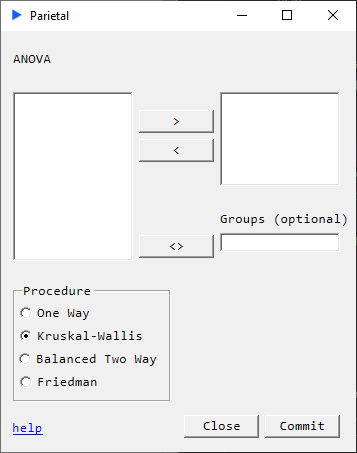#### Description#

Nonparametric test to compare means of multiple samples.

$H_{0}$ : the central values of data distributions are equal
$H_{a}$ : at least one of the central values is different

#### Assumptions:#

• Continuous distributions
• Independent samples

#### Returns#

SourceSSDFMSFP
$Treatment\hspace{1mm}$$SST\hspace{3mm}$$k-1\hspace{3mm}$$SST/(k-1)\hspace{3mm}$$MST/MSE\hspace{3mm}$$p Error\hspace{1mm}$$SSE\hspace{3mm}$$N-k\hspace{3mm}$$SSE/(N-k)\hspace{3mm}$
$Total\hspace{1mm}$$SS\hspace{3mm}$$N-1\hspace{3mm}$

## Balanced Two Way ANOVA##### Description#

Determines the difference between means in unrelated groups across two factors. This is an extension of the one-way ANOVA where there is only one factor.

$H_{0}: \begin{cases} \text{population means of the factor A are equal} \\ \text{population means of the factor B are equal} \\ \text{no interaction} \end{cases}$

#### Assumptions:#

• Residuals must be normally distributed for each combination of levels of the independent variables
• Variances are homogenous
• Independent samples

#### Returns#

$$\scriptscriptstyle \begin{matrix} Source & SS & DF & MS & F & P \\ Factor A & SSA & k-1 & SSA/df_{A} & MS_{A}/MS_{E} & p \\ Factor B & SSB & j-1 & SSB/df_{B} & MS_{B}/MS_{E} & \\ Interaction & SSAB & (k-1)(j-1) & SSAB/(df_{A}df_{B}) & MS_{AB}/MS_{E} & \\ Error & SSE & kj(r-1) & SSE/df_{E} \\ Total & SST & rjk-1 \end{matrix}$$

## Friedman#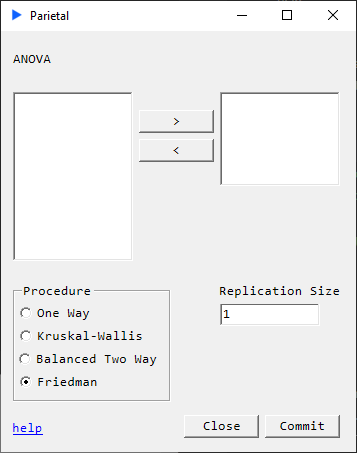#### Description#

Nonparametric alternative to repeated measures two-way ANOVA.

$H_{0}$: the distributions are equal across repeated measures
$H_{a}$: the distributions are different across repeated measures

• ANOVA table